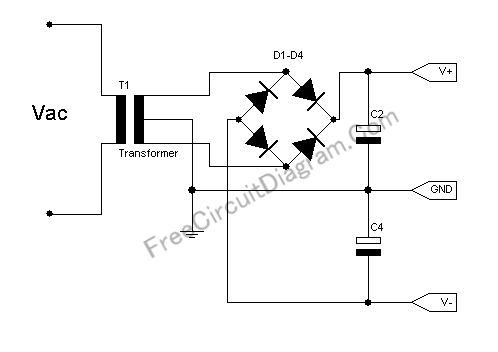# Basic Symmetric Power SupplyThe circuit below is the basic for standard symmetrical power supply. If your circuit need high current then this circuit is suitable. Here you can see the circuit’s schematic diagram.You need a center-tapped transformer to build this symmetric power supply. This power supply circuit is widely used in op-amp application, as well as in high-rated power amplifier. If you don’t have a center-tapped transformer and you need only small current for your circuit then many alternative  options for symmetric power supply can be found here ( symmetric power supply 1), here (symmetric power supply 2), and here (symmetric power supply 3).